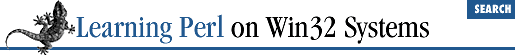#Chapter 5Hashes## 5.5 Hash Slices

Like an array variable (or list literal), a hash can be sliced to access a collection of elements instead of just one element at a time. For example, consider the bowling scores set individually:

```\$score{"fred"} = 205;
\$score{"barney"} = 195;
\$score{"dino"} = 30;```

This collection seems rather redundant, and in fact can be shortened to:

```(\$score{"fred"},\$score{"barney"},\$score{"dino"}) =
(205,195,30);```

But even these seem redundant. Let's use a hash slice :

`@score{"fred","barney","dino"} = (205,195,30);`

There. Much shorter. We can use a hash slice with variable interpolation as well:

```@players = qw(fred barney dino);
print "scores are: @score{@players}\n";```

Hash slices can also be used to merge a smaller hash into a larger one. In this example, the smaller hash takes precedence in the sense that if there are duplicate keys, the value from the smaller hash is used:

`@league{keys %score} = values %score;`

Here, the values of `%score` are merged into the `%league` hash. This operation is equivalent to the much slower operation:

`%league = (%league, %score); # merge %score into %league`